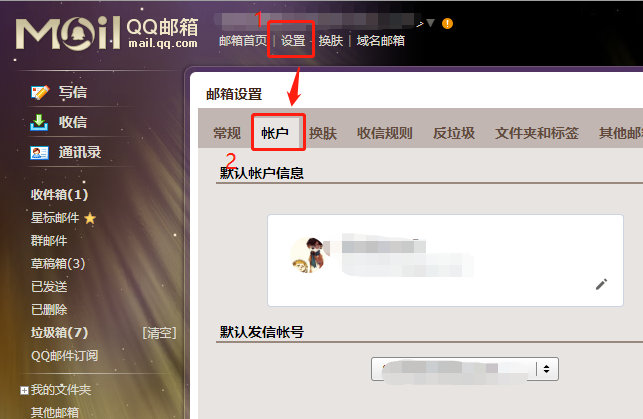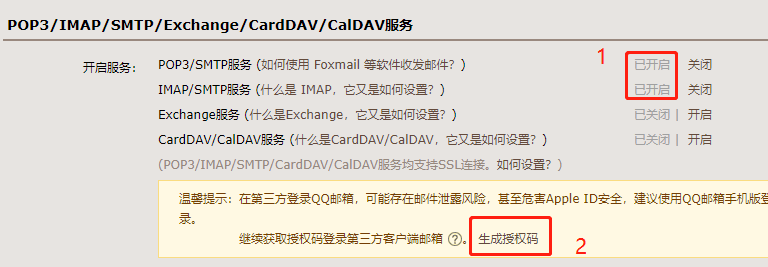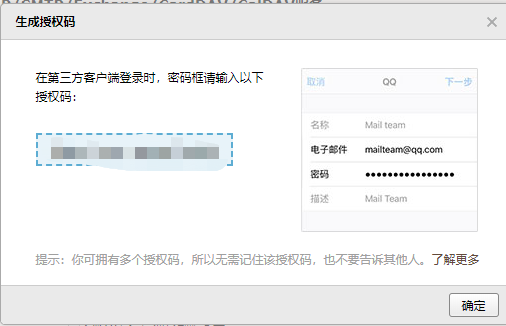# Python实战之自动发送邮件的实现

## 1.开启SMTP服务## 2.准备

(可选1) 如果你用Python的目的是数据分析，可以直接安装Anaconda，它内置了Python和pip.

(可选2) 此外，推荐大家用VSCode编辑器，它有许多的优点

1. Windows 环境 打开 Cmd (开始-运行-CMD)。

2. MacOS 环境 打开 Terminal (command+空格输入Terminal)。

3. 如果你用的是 VSCode编辑器 或 Pycharm，可以直接使用界面下方的Terminal.

`pip install yagmail`

## 3.编写脚本

```yag = yagmail.SMTP(
host='smtp.qq.com', user='你的邮箱',
).send(["发送对象"], "主题", "内容")```

```import time
import yagmail

class Mail:
"""
邮件相关类
"""

"""
发送邮件

Arguments:
msg {str} -- 邮件正文
title {str} -- 邮件标题
"""

yag = yagmail.SMTP(
host='smtp.qq.com', user='你的邮箱',
)

try:
print("邮件发送成功")

except BaseException as e:
print(e)
print("Error: 无法发送邮件")```

```import time
import yagmail

class Mail:
"""
邮件相关类
"""

def log(self, content):
now_time = time.strftime(
"%Y-%m-%d %H:%M:%S", time.localtime()
)
print(f'{now_time}: {content}')```

```import time
import yagmail

class Mail:
"""
邮件相关类
"""

def log(self, content):
now_time = time.strftime(
"%Y-%m-%d %H:%M:%S", time.localtime()
)
print(f'{now_time}: {content}')

"""
发送邮件

Arguments:
msg {str} -- 邮件正文
title {str} -- 邮件标题
"""

yag = yagmail.SMTP(
host='smtp.qq.com', user='你的邮箱',
)

try:
self.log("邮件发送成功")

except BaseException as e:
print (e)
self.log("Error: 无法发送邮件")```

```# PS D:\CODE\stock\api> python .\sendmail_yagmail.py
2021-06-21 00:00:00: 邮件发送成功```

## 4.小例子

```import time
import requests
from sendmail import Mail

while True:
response = requests.get('https://pythondict.com')

# 根据状态码判断网站是否正常
if response.status_code != 200:
Mail().sendmail(
'哥, pythondict挂了', 'Python实用宝典网站异常监控', ['你的邮箱']
)

time.sleep(600)```

`nohup python test.py &`

0
0

0 条回复（回复会通过微信通知作者）

（微信扫码即可登录，无需注册）